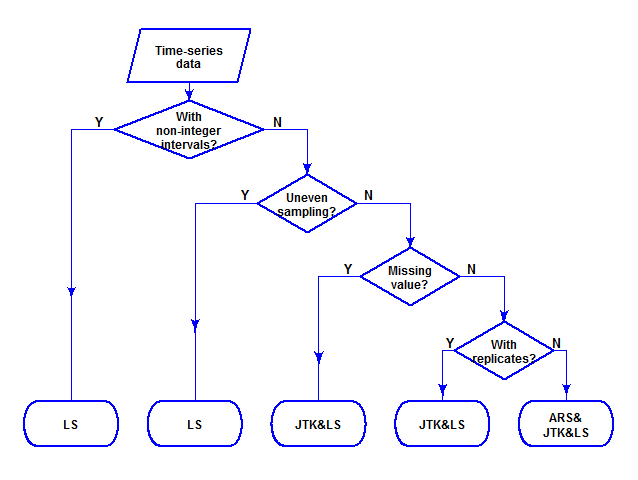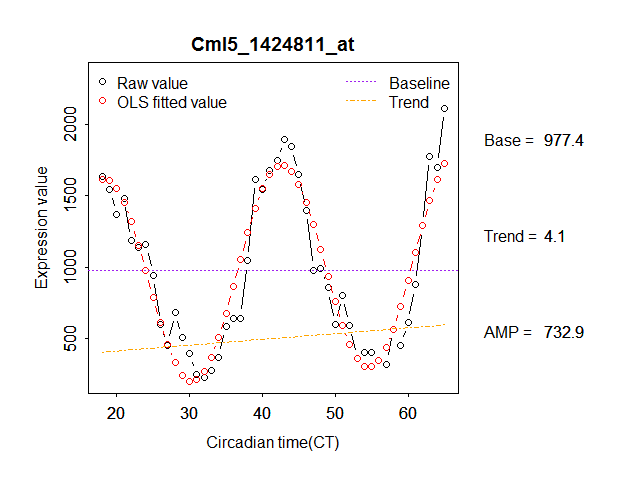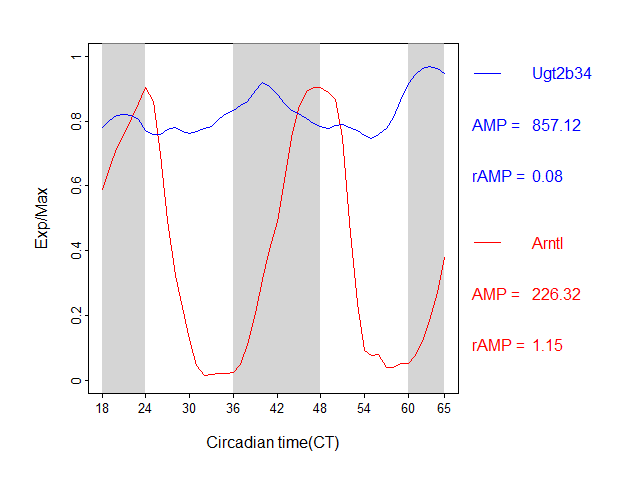The MetaCycle package is mainly used for detecting rhythmic signals from large scale time-series data. Depending on features of each time-series data, MetaCycle incorporates ARSER(ARS), JTK_CYCLE(JTK), and Lomb-Scargle(LS) properly for periodic signal detection, and it could also output integrated analysis results if required.

This vignette introduces implementation of method selection and integration steps of MetaCycle, which are not detaily explained in help files. For looking at how to use two main functions–meta2d and meta3d of this package, please see ‘Examples part’ of each function’s help file.

The MetaCycle source code will be available on github later.

## Time-series datasets

### Two main categories

A typical time-series dataset from a non-human organism is a two-dimensional matrix. Each row indicates one molecular’s profile along time, and all molecule at any one time point are detected from the same sample. It is usually not necessary to keep track of which individual organism does a sample come from. For easily explanation, we named this kind of dataset as 2D time-series dataset. Take the time-series transcriptome dataset from mouse liver as an example.

library(MetaCycle)
head(cycMouseLiverRNA[,1:5])
##                geneName       CT18       CT19       CT20       CT21
## 1 Hist1h1c_1416101_a_at 2700.33576 2394.28784 2298.08895 2097.18536
## 2      Fkbp5_1448231_at   60.46103   56.37786  109.55954   53.22913
## 3    Nr1h3_1450444_a_at  438.05912  462.95678  472.93666  451.21432
## 4     Avpr1a_1418603_at  185.53679  209.94027  371.56557  246.81055
## 5       Lipg_1421262_at   56.38897   51.47247   45.50319   33.26548
## 6       Scap_1433520_at  910.17842  913.61711  797.53662  855.48581

For time-series datasets from human, it is usually essential to keep track of the individual information about each sample. Except one matrix stores experimental values of detected molecule from all samples, another matrix is necessary to store individual information of each sample. This kind of dataset is named as 3D time-series dataset. For example, a time-series dataset from human blood is shown as below.

The individual information matrix:

set.seed(100)
row_index <- sample(1:nrow(cycHumanBloodDesign), 4)
cycHumanBloodDesign[row_index,]
##     sample_library subject            group time_hoursawake
## 123      GSM969119  AF0205   SleepExtension            16.5
## 103      GSM969058  AF0164   SleepExtension            16.5
## 220      GSM968870  AF0010 SleepRestriction            34.5
## 23       GSM968874  AF0033   SleepExtension            28.5

The corresponding experimental values:

sample_id <- cycHumanBloodDesign[row_index,1]
head(cycHumanBloodData[,c("ID_REF", sample_id)])
##         ID_REF GSM969119 GSM969058 GSM968870 GSM968874
## 1 FBXL16_24786 11.492014 11.992029 10.024246 11.182840
## 2   PLD6_33164  8.690105  8.631630  8.962610  8.749021
## 3   MPZL1_7604  7.510391  7.283362  7.746354  7.313027
## 4    LRG1_9183 11.604538 10.870134 13.155444 11.442670
## 5  NELL2_31679 10.602785 10.935570  9.948586 10.308829
## 6   GHRL_21324  9.437338  9.635632 10.741739 10.007841

A 3D time-series dataset could be divided into multiple 2D time-series datasets, and all experimental values for an individual under the same treatment are in one 2D time-series dataset. For example, we could extracted all experimental values from “AF0004” under “SleepExtension” into one 2D time-series dataset.

group_index <- which(cycHumanBloodDesign[, "group"] == "SleepExtension")
cycHumanBloodDesignSE <- cycHumanBloodDesign[group_index,]
sample_index <- which(cycHumanBloodDesignSE[, "subject"] == "AF0004")
sample_AF0004 <- cycHumanBloodDesignSE[sample_index, "sample_library"]
cycHumanBloodDataSE_AF0004 <- cycHumanBloodData[, c("ID_REF", sample_AF0004)]
head(cycHumanBloodDataSE_AF0004)
##         ID_REF GSM968833 GSM968834 GSM968835 GSM968836 GSM968837 GSM968838
## 1 FBXL16_24786  9.463928  9.577096  9.555228  9.762367  9.632398  9.300298
## 2   PLD6_33164  8.871161  8.904331  8.891287  8.976349  9.120056  8.780435
## 3   MPZL1_7604  6.980270  7.038884  7.029386  6.701037  6.445718  6.612718
## 4    LRG1_9183 10.872974 11.069575 11.717534 10.648445 10.551989 10.969216
## 5  NELL2_31679 10.201968 10.286969 10.351848 10.664220 10.885527 10.370518
## 6   GHRL_21324 10.281647 10.340997 10.375283  9.965949 10.244704 10.514198
##   GSM968839 GSM968840 GSM968841 GSM968842
## 1  9.232959  8.457440  8.952968  9.009024
## 2  8.781804  8.449115  8.600893  8.703119
## 3  6.976794  7.726147  7.914560  7.049164
## 4 10.806980 12.362691 11.848146 11.093384
## 5 10.127192  9.255606  9.618674  9.941711
## 6 10.519501 11.161733 10.672796 10.321841

### Detail types of 2D time-series dataset

One kind of usual 2D time-series dataset is evenly sampled once at each time point, and the interval between neighbour time points is integer. Not all datasets are as simple as this. There are datasets with replicate samples, or with missing values, or un-evenly sampled, or sampled with a non-integer interval. Examples of these types of dataset are shown in the below table.

Data Type Point 1 Point 2 Point 3 Point 4 Point 5 Point 6
The usual data CT0 CT4 CT8 CT12 CT16 CT20
With missing value CT0 NA CT8 CT12 CT16 CT20
With replicates CT0 CT0 CT8 CT8 CT16 CT16
With un-even interval CT0 CT2 CT8 CT10 CT16 CT20
With non-integer interval CT0 CT4.5 CT9 CT13.5 CT18 CT22.5

Of course, some datasets may seem combination of two or more of above types of data.

Data Type Point 1 Point 2 Point 3 Point 4 Point 5 Point 6
With replicates and missing value CT0 CT0 CT8 NA CT16 CT16
With un-even interval and replicates CT0 CT2 CT2 CT10 CT16 CT20

## Method selection

The meta2d function in MetaCycle is designed to analyze 2D time-series datasets, and it could automatically select proper method to analyze different types of input datasets. The implementation strategy used for meta2d is shown in the flow chart (drawn with “diagram” package).For analyzing 3D time-series dataset, meta3d function in MetaCycle is suggested. It firstly divides the input dataset into multiple 2D time-series datasets based on individual information, and then use the defined method through calling meta2d to analyze each divided dataset.

## Integration

In addition to selecting proper methods to analyze different kinds of datasets, MetaCycle could also output integrated results. In detail, meta2d integrates analysis results from multiple methods and meta3d integrates analysis results from multiple individuals.

### Pvalue

Fisher’s method is implemented in both meta2d and meta3d for integrating multiple p-values. The below formula is used to combine multiple p-values into one test statistic (X2).

$X^2_{2k} \sim -2\sum_{i=1}^k ln(p_i)$

X2 has a chi-squared distribution with 2k degrees of freedom (k is the number of p-values), when all the null hypotheses are true, and each p-value is independent. The combined p-value is determined by the p-value of X2.

### Period and phase

The integrated period from MetaCycle is an arithmetic mean value of multiple periods, while phase integration based on mean of circular quantities is implemented in meta2d and meta3d. The detail steps are as below.

• convert phase values to polar coordinates $$\alpha_j$$
• convert polar coordinates to cartesian coordinates ($$cos\alpha_j$$, $$sin\alpha_j$$)
• compute the arithmetic mean of these points and its corresponding polar coordinate $$\bar{\alpha}$$ $\bar{\alpha} = atan2(\frac{\sum_{j=1}^n sin\alpha_j}{n}, \frac{\sum_{j=1}^n cos\alpha_j}{n})$
• convert the resulting polar coordinate to a integrated phase value
# given three phases
pha <- c(0.9, 0.6, 23.6)
# their corresponding periods
per <- c(23.5, 24, 24.5)
# mean period length
per_mean <- mean(per)
# covert to polar coordinate
polar <- 2*pi*pha/per
# get averaged ploar coordinate
polar_mean <- atan2(mean(sin(polar)), mean(cos(polar)))
# get averaged phase value
pha_mean <- per_mean*polar_mean/(2*pi)
pha_mean
##  0.2159827

### Amplitude calculation

meta2d recalculates the amplitude with following model:

$Y_i = B + TRE*(t_i - \frac{\sum_{i=1}^n t_i}{n}) + A*cos(2*\pi*\frac{t_i - PHA}{PER})$

where $$Y_i$$ is the observed value at time $$t_i$$; B is baseline level of the time-series profile; TRE is trend level of the time-series profile; A is the amplitude of the waveform. PHA and PER are integrated period and phase mentioned above. In this model, only B, TRE and A are unknown parameters, which could be calculated with ordinary least square (OLS) method. The baseline and trend level are explained in the below example.In addition, meta2d also output a relative amplitude value (rAMP), which could be easily taken as the ratio between amplitude and baseline (if |B| >= 1). The amplitude value is associated with the general expression level, which indicates highly expressed genes may always have larger amplitude than lowly expressed genes. The rAMP may be used to compare the amplitude values among genes with different expression levels. For example, Ugt2b34 has a larger amplitude than Arntl, but its rAMP is smaller than Arntl.Based on the calculated baseline, amplitude and relative amplitude values by meta2d, meta3d calculates the corresponding integrated values with arithmetic mean of multiple individuals in each group.